# Snake Game in C

• Difficulty Level : Easy
• Last Updated : 10 Feb, 2021

In this article, the task is to implement a basic Snake Game. Below given some functionalities of this game:

• The snake is represented with a 0(zero) symbol.
• The fruit is represented with an *(asterisk) symbol.
• The snake can move in any direction according to the user with the help of the keyboard (W, A, S, D keys).
• When the snake eats a fruit the score will increase by 10 points.
• The fruit will generate automatically within the boundaries.
• Whenever the snake will touch the boundary the game is over.

Steps to create this game:

Want to learn from the best curated videos and practice problems, check out the C++ Foundation Course for Basic to Advanced C++ and C++ STL Course for the language and STL. To complete your preparation from learning a language to DS Algo and many more, please refer Complete Interview Preparation Course.
• There will be four user-defined functions.
• Build a boundary within which the game will be played.
• The fruits are generated randomly.
• Then increase the score whenever the snake eats a fruit.

The user-defined functions created in this program are given below:

• Draw(): This function creates the boundary in which the game will be played.
• Setup(): This function will set the position of the fruit within the boundary.
• Input(): This function will take the input from the keyboard.
• Logic(): This function will set the movement of the snake.

Built-in functions used:

• kbhit(): This function in C is used to determine if a key has been pressed or not. To use this function in a program include the header file conio.h. If a key has been pressed, then it returns a non-zero value otherwise it returns zero.
• rand(): The rand() function is declared in stdlib.h. It returns a random integer value every time it is called.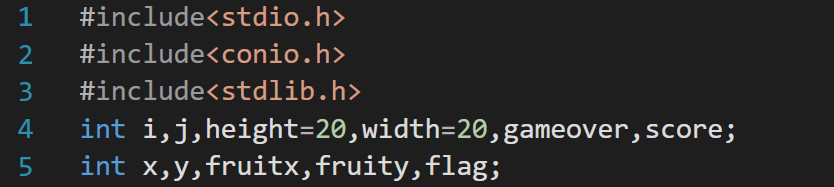Draw(): This function is responsible to build the boundary within which the game will be played.

Below is the C program to build the outline boundary using draw():

## C

 `// C program to build the outline``// boundary using draw()``#include ``#include ``int` `i, j, height = 30;``int` `width = 30, gameover, score;`` ` `// Function to draw a boundary``void` `draw()``{``    ``// system("cls");``    ``for` `(i = 0; i < height; i++) {``        ``for` `(j = 0; j < width; j++) {``            ``if` `(i == 0 || i == width - 1 || j == 0``                ``|| j == height - 1) {``                ``printf``(``"#"``);``            ``}``            ``else` `{``                ``printf``(``" "``);``            ``}``        ``}``        ``printf``(``"\n"``);``    ``}``}`` ` `// Driver Code``int` `main()``{``    ``// Function Call``    ``draw();`` ` `    ``return` `0;``}`
Output:
```##############################
#                            #
#                            #
#                            #
#                            #
#                            #
#                            #
#                            #
#                            #
#                            #
#                            #
#                            #
#                            #
#                            #
#                            #
#                            #
#                            #
#                            #
#                            #
#                            #
#                            #
#                            #
#                            #
#                            #
#                            #
#                            #
#                            #
#                            #
#                            #
##############################
```

setup():nThisbfunction is used to write the code to generate the fruit within the boundary using rand() function.

• Using rand()%20 because the size of the boundary is length = 20 and width = 20 so the fruit will generate within the boundary.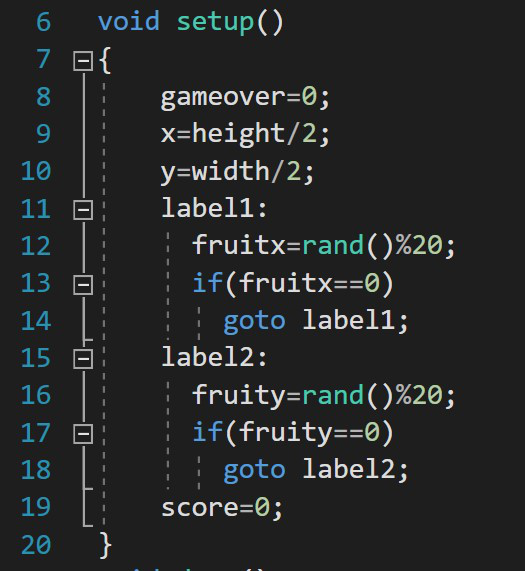Input(): In this function, the programmer writes the code to take the input from the keyboard (W, A, S, D, X keys).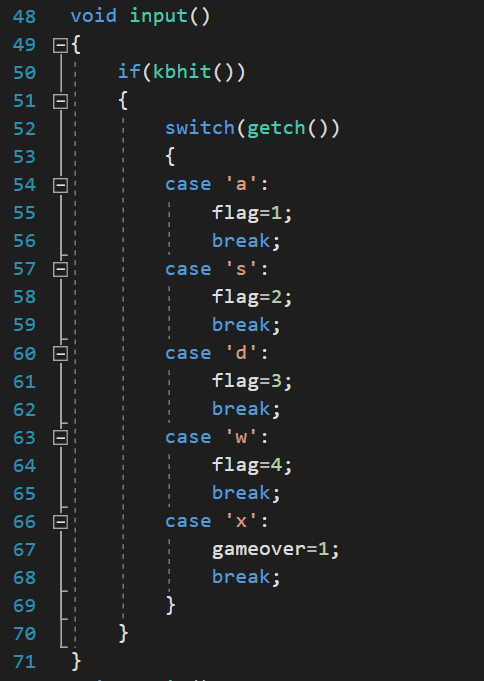logic(): Here, write all the logic for this program like for the movement of the snake, for increasing the score, when the snake will touch the boundary the game will be over, to exit the game and the random generation of the fruit once the snake will eat the fruit.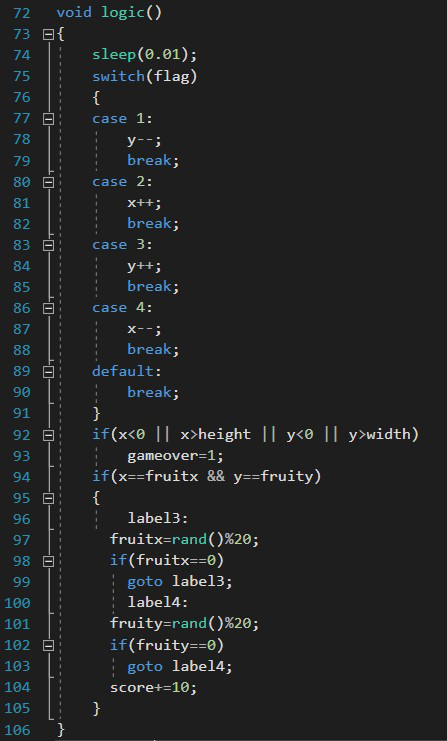sleep(): This function in C is a function that delays the program execution for the given number of seconds. In this code sleep() is used to slow down the movement of the snake so it will be easy for the user to play.

main(): From the main() function the execution of the program starts. It calls all the functions.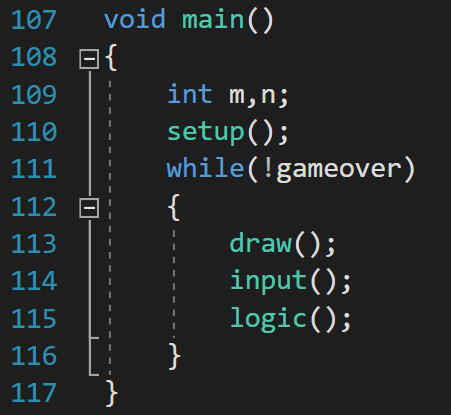Below is the C program to build the complete snake game:

## C

 `// C program to build the complete``// snake game``#include ``#include ``#include ``#include `` ` `int` `i, j, height = 20, width = 20;``int` `gameover, score;``int` `x, y, fruitx, fruity, flag;`` ` `// Function to generate the fruit``// within the boundary``void` `setup()``{``    ``gameover = 0;`` ` `    ``// Stores height and width``    ``x = height / 2;``    ``y = width / 2;``label1:``    ``fruitx = ``rand``() % 20;``    ``if` `(fruitx == 0)``        ``goto` `label1;``label2:``    ``fruity = ``rand``() % 20;``    ``if` `(fruity == 0)``        ``goto` `label2;``    ``score = 0;``}`` ` `// Function to draw the boundaries``void` `draw()``{``    ``system``(``"cls"``);``    ``for` `(i = 0; i < height; i++) {``        ``for` `(j = 0; j < width; j++) {``            ``if` `(i == 0 || i == width - 1``                ``|| j == 0``                ``|| j == height - 1) {``                ``printf``(``"#"``);``            ``}``            ``else` `{``                ``if` `(i == x && j == y)``                    ``printf``(``"0"``);``                ``else` `if` `(i == fruitx``                         ``&& j == fruity)``                    ``printf``(``"*"``);``                ``else``                    ``printf``(``" "``);``            ``}``        ``}``        ``printf``(``"\n"``);``    ``}`` ` `    ``// Print the score after the``    ``// game ends``    ``printf``(``"score = %d"``, score);``    ``printf``(``"\n"``);``    ``printf``(``"press X to quit the game"``);``}`` ` `// Function to take the input``void` `input()``{``    ``if` `(kbhit()) {``        ``switch` `(getch()) {``        ``case` `'a'``:``            ``flag = 1;``            ``break``;``        ``case` `'s'``:``            ``flag = 2;``            ``break``;``        ``case` `'d'``:``            ``flag = 3;``            ``break``;``        ``case` `'w'``:``            ``flag = 4;``            ``break``;``        ``case` `'x'``:``            ``gameover = 1;``            ``break``;``        ``}``    ``}``}`` ` `// Function for the logic behind``// each movement``void` `logic()``{``    ``sleep(0.01);``    ``switch` `(flag) {``    ``case` `1:``        ``y--;``        ``break``;``    ``case` `2:``        ``x++;``        ``break``;``    ``case` `3:``        ``y++;``        ``break``;``    ``case` `4:``        ``x--;``        ``break``;``    ``default``:``        ``break``;``    ``}`` ` `    ``// If the game is over``    ``if` `(x < 0 || x > height``        ``|| y < 0 || y > width)``        ``gameover = 1;`` ` `    ``// If snake reaches the fruit``    ``// then update the score``    ``if` `(x == fruitx && y == fruity) {``    ``label3:``        ``fruitx = ``rand``() % 20;``        ``if` `(fruitx == 0)``            ``goto` `label3;`` ` `    ``// After eating the above fruit``    ``// generate new fruit``    ``label4:``        ``fruity = ``rand``() % 20;``        ``if` `(fruity == 0)``            ``goto` `label4;``        ``score += 10;``    ``}``}`` ` `// Driver Code``void` `main()``{``    ``int` `m, n;`` ` `    ``// Generate boundary``    ``setup();`` ` `    ``// Until the game is over``    ``while` `(!gameover) {`` ` `        ``// Function Call``        ``draw();``        ``input();``        ``logic();``    ``}``}`

Output: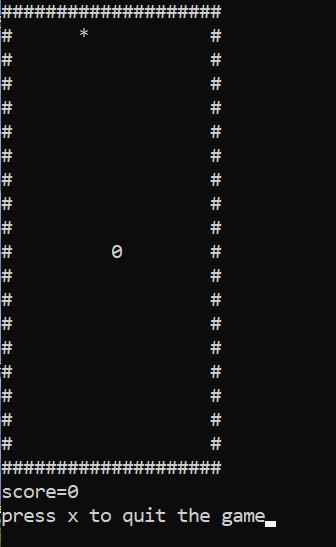Demonstration:

My Personal Notes arrow_drop_up